# 时间序列预测的7种方法

## 数据准备

import pandas as pd
import numpy as np
import matplotlib.pyplot as plt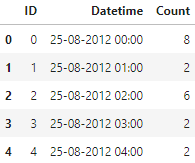• 从 2012 年 8 月- 2013 年 12 月的数据中构造一个数据集。
• 创建 train and test 文件用于建模。前 14 个月（ 2012 年 8 月- 2013 年 10 月）用作训练数据，后两个月（2013 年 11 月 – 2013 年 12 月）用作测试数据。
• 以每天为单位聚合数据集。
import pandas as pd
import matplotlib.pyplot as plt

# Subsetting the dataset
# Index 11856 marks the end of year 2013

# Creating train and test set
# Index 10392 marks the end of October 2013
train = df[0:10392]
test = df[10392:]

# Aggregating the dataset at daily level
df['Timestamp'] = pd.to_datetime(df['Datetime'], format='%d-%m-%Y %H:%M')
df.index = df['Timestamp']
df = df.resample('D').mean()

train['Timestamp'] = pd.to_datetime(train['Datetime'], format='%d-%m-%Y %H:%M')
train.index = train['Timestamp']
train = train.resample('D').mean()

test['Timestamp'] = pd.to_datetime(test['Datetime'], format='%d-%m-%Y %H:%M')
test.index = test['Timestamp']
test = test.resample('D').mean()

#Plotting data
train.Count.plot(figsize=(15,8), title= 'Daily Ridership', fontsize=14)
test.Count.plot(figsize=(15,8), title= 'Daily Ridership', fontsize=14)
plt.show()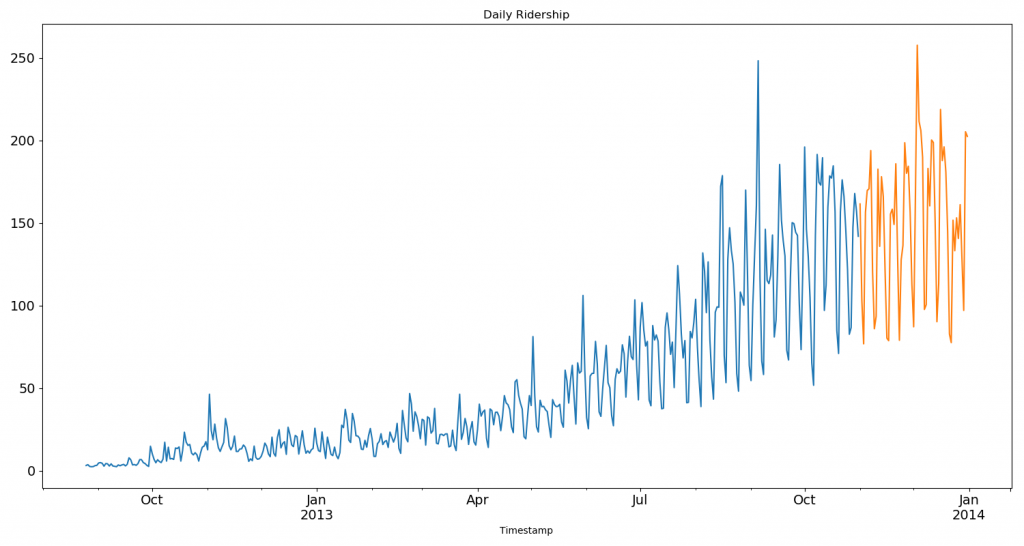## 方法1：朴素法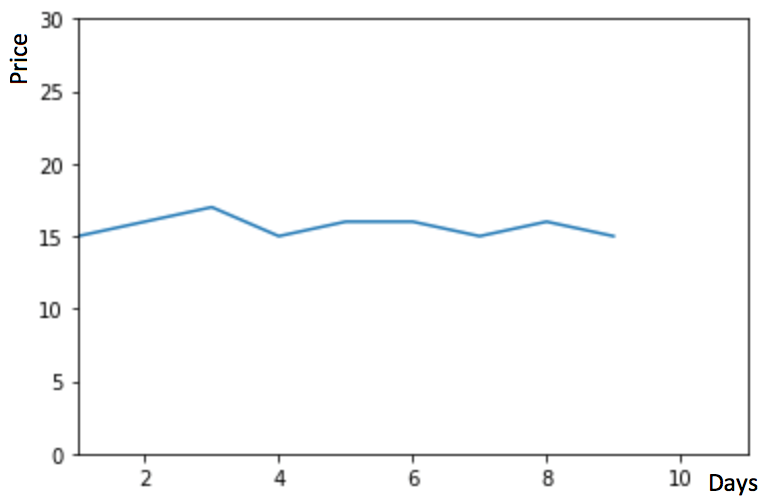$$\hat{y}_{t+1} = y_t$$

dd = np.asarray(train['Count'])
y_hat = test.copy()
y_hat['naive'] = dd[len(dd) - 1]
plt.figure(figsize=(12, 8))
plt.plot(train.index, train['Count'], label='Train')
plt.plot(test.index, test['Count'], label='Test')
plt.plot(y_hat.index, y_hat['naive'], label='Naive Forecast')
plt.legend(loc='best')
plt.title("Naive Forecast")
plt.show()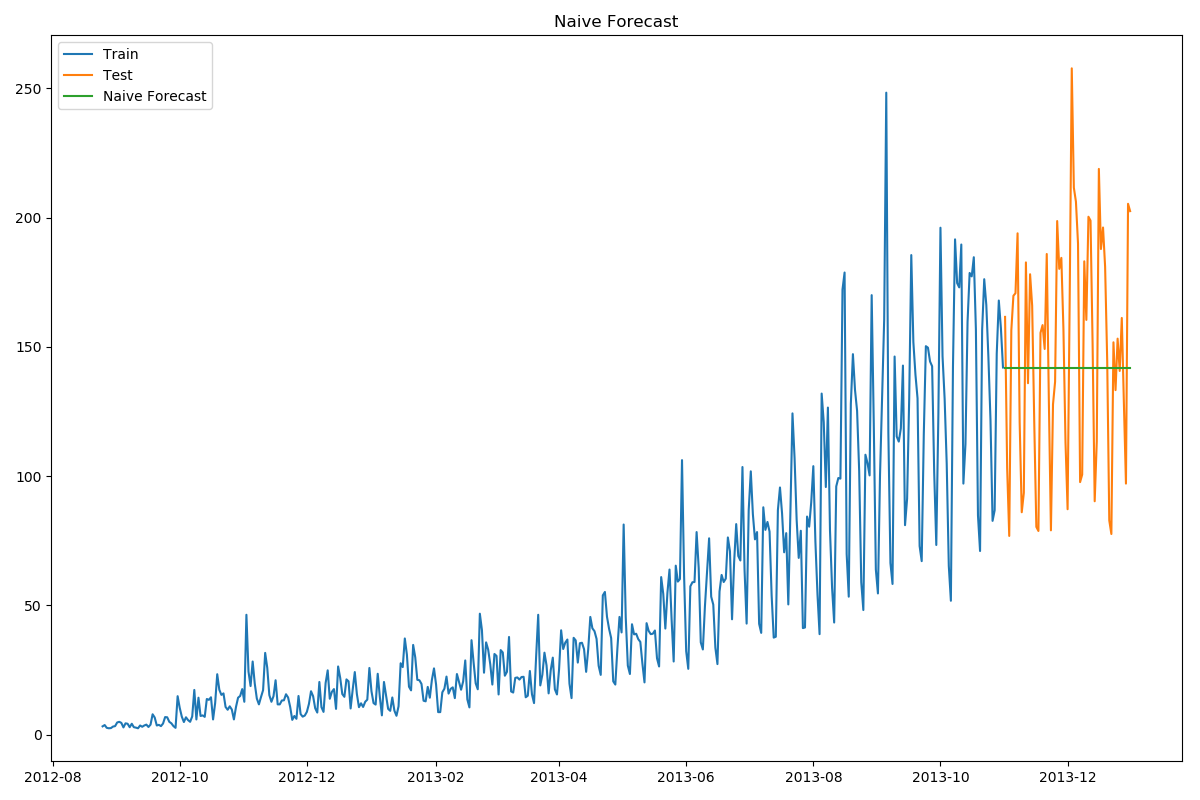from sklearn.metrics import mean_squared_error
from math import sqrt

rms = sqrt(mean_squared_error(test['Count'], y_hat['naive']))
print(rms)


## 方法2：简单平均法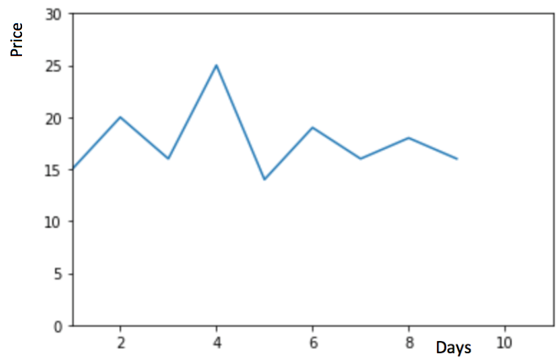$$\hat{y}_{x+1} = \frac{1}{x}\sum_{i=1}^{x}y_{i}$$

y_hat_avg = test.copy()
y_hat_avg['avg_forecast'] = train['Count'].mean()
plt.figure(figsize=(12,8))
plt.plot(train['Count'], label='Train')
plt.plot(test['Count'], label='Test')
plt.plot(y_hat_avg['avg_forecast'], label='Average Forecast')
plt.legend(loc='best')
plt.show()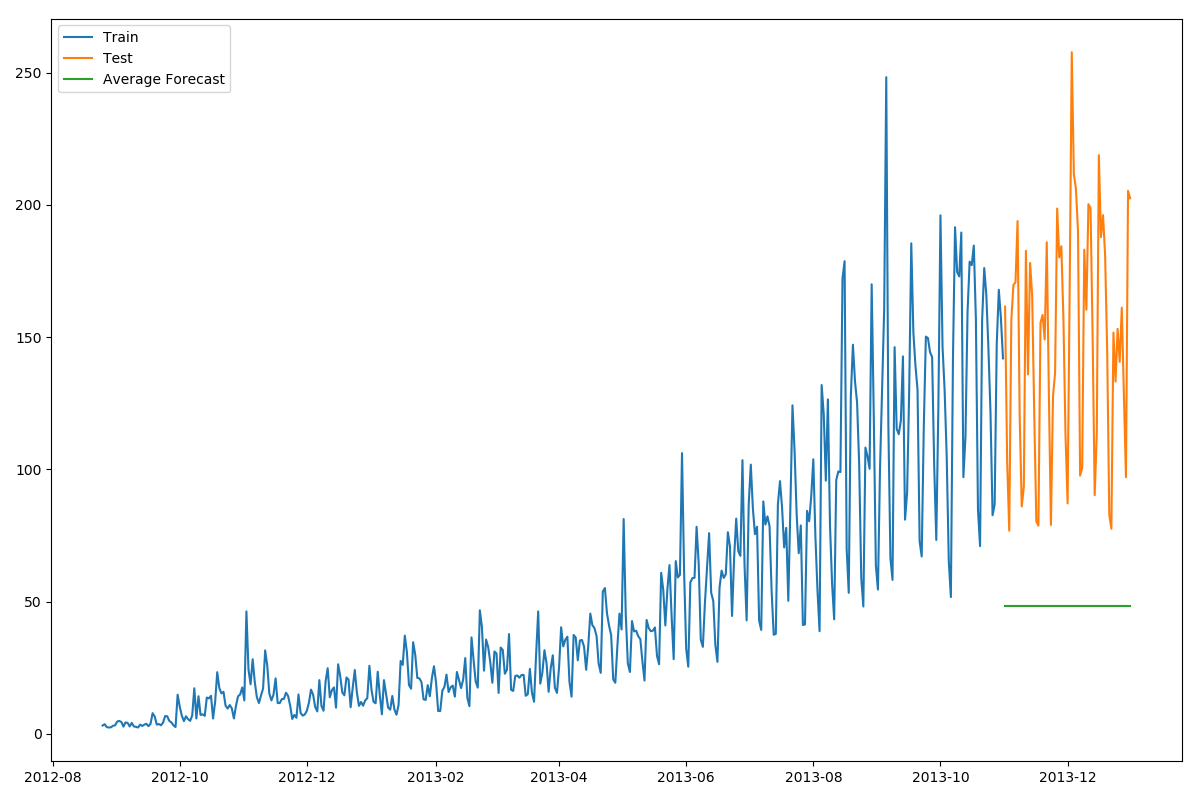from sklearn.metrics import mean_squared_error
from math import sqrt

rms = sqrt(mean_squared_error(test['Count'], y_hat_avg['avg_forecast']))
print(rms)

## 方法3：移动平均法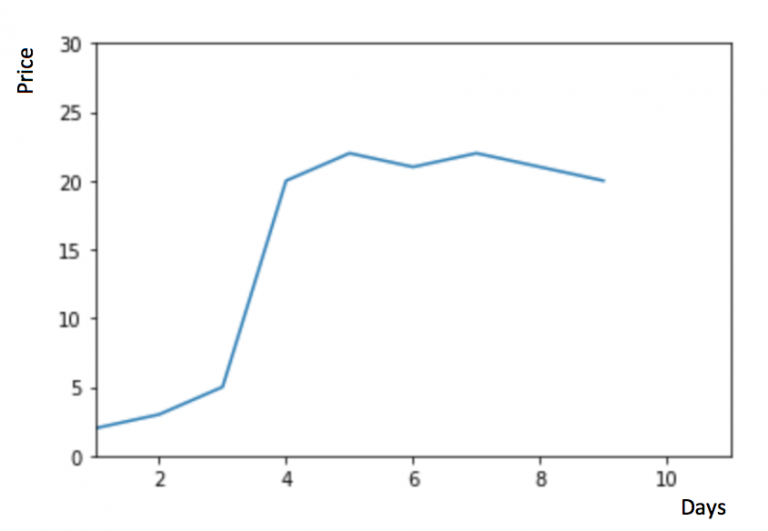$$\hat{y}_{l} = \frac{1}{p}(y_{i-1}+y_{i-2}+y_{i-3}+…+y_{i-p})$$

y_hat_avg = test.copy()
y_hat_avg['moving_avg_forecast'] = train['Count'].rolling(60).mean().iloc[-1]
plt.figure(figsize=(16,8))
plt.plot(train['Count'], label='Train')
plt.plot(test['Count'], label='Test')
plt.plot(y_hat_avg['moving_avg_forecast'], label='Moving Average Forecast')
plt.legend(loc='best')
plt.show()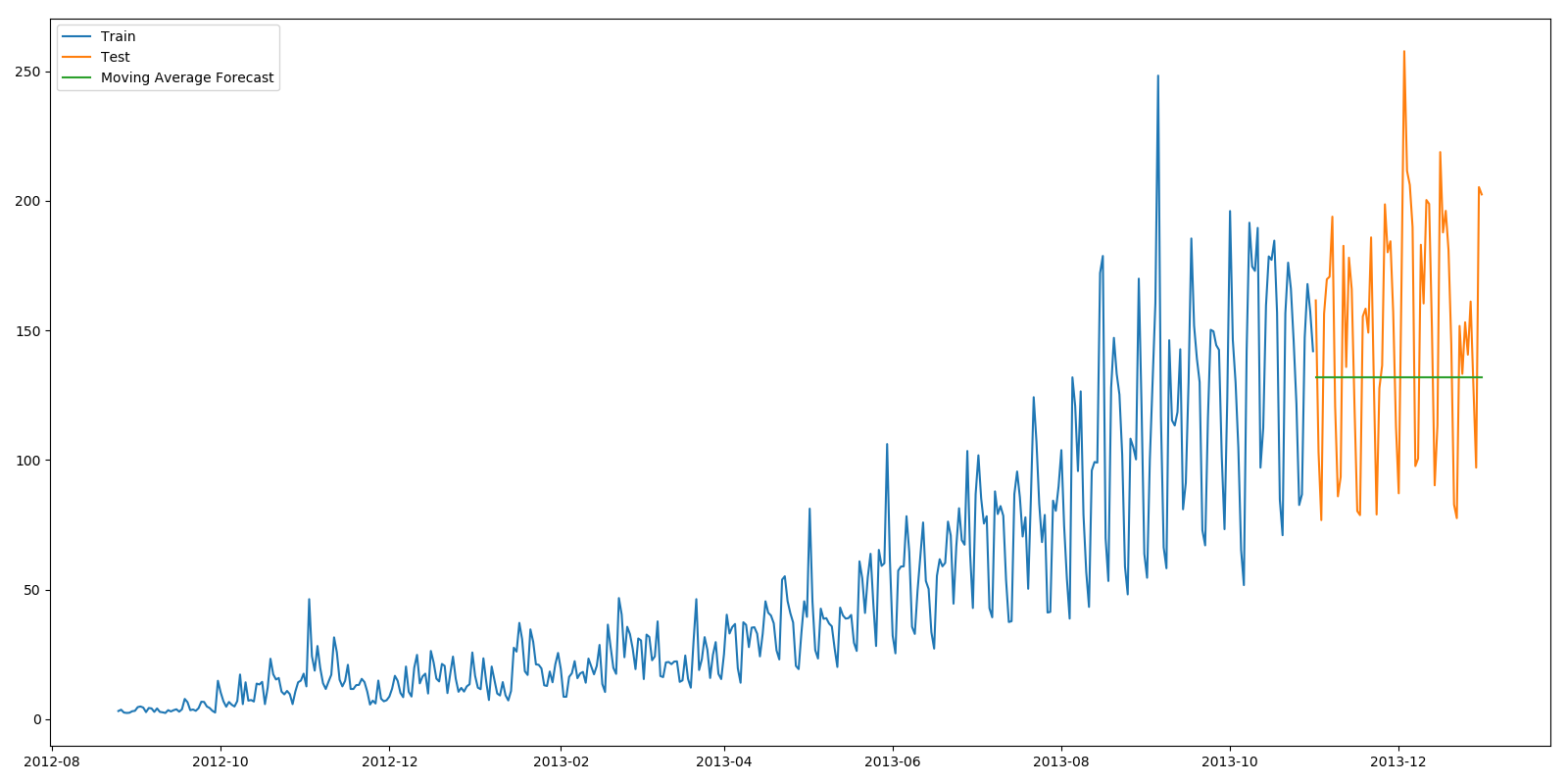from sklearn.metrics import mean_squared_error
from math import sqrt

rms = sqrt(mean_squared_error(test['Count'], y_hat_avg['moving_avg_forecast']))
print(rms)

$$\hat{y}_{l} = \frac{1}{m}(w_1*y_{i-1}+w_2*y_{i-2}+w_3*y_{i-3}+…+w_m*y_{i-m})$$

## 方法4：简单指数平滑法

$$\hat{y}_{T+1|T}=\alpha y_T+\alpha(1-\alpha)y_{T-1}+\alpha(1-\alpha)^{2}y_{T-2}+…$$

$$\hat{y}_{t+1|t}=\alpha y_t+(1-\alpha)\hat{y}_{t-1|t}$$

from statsmodels.tsa.api import SimpleExpSmoothing

y_hat_avg = test.copy()
fit = SimpleExpSmoothing(np.asarray(train['Count'])).fit(smoothing_level=0.6, optimized=False)
y_hat_avg['SES'] = fit.forecast(len(test))
plt.figure(figsize=(16, 8))
plt.plot(train['Count'], label='Train')
plt.plot(test['Count'], label='Test')
plt.plot(y_hat_avg['SES'], label='SES')
plt.legend(loc='best')
plt.show()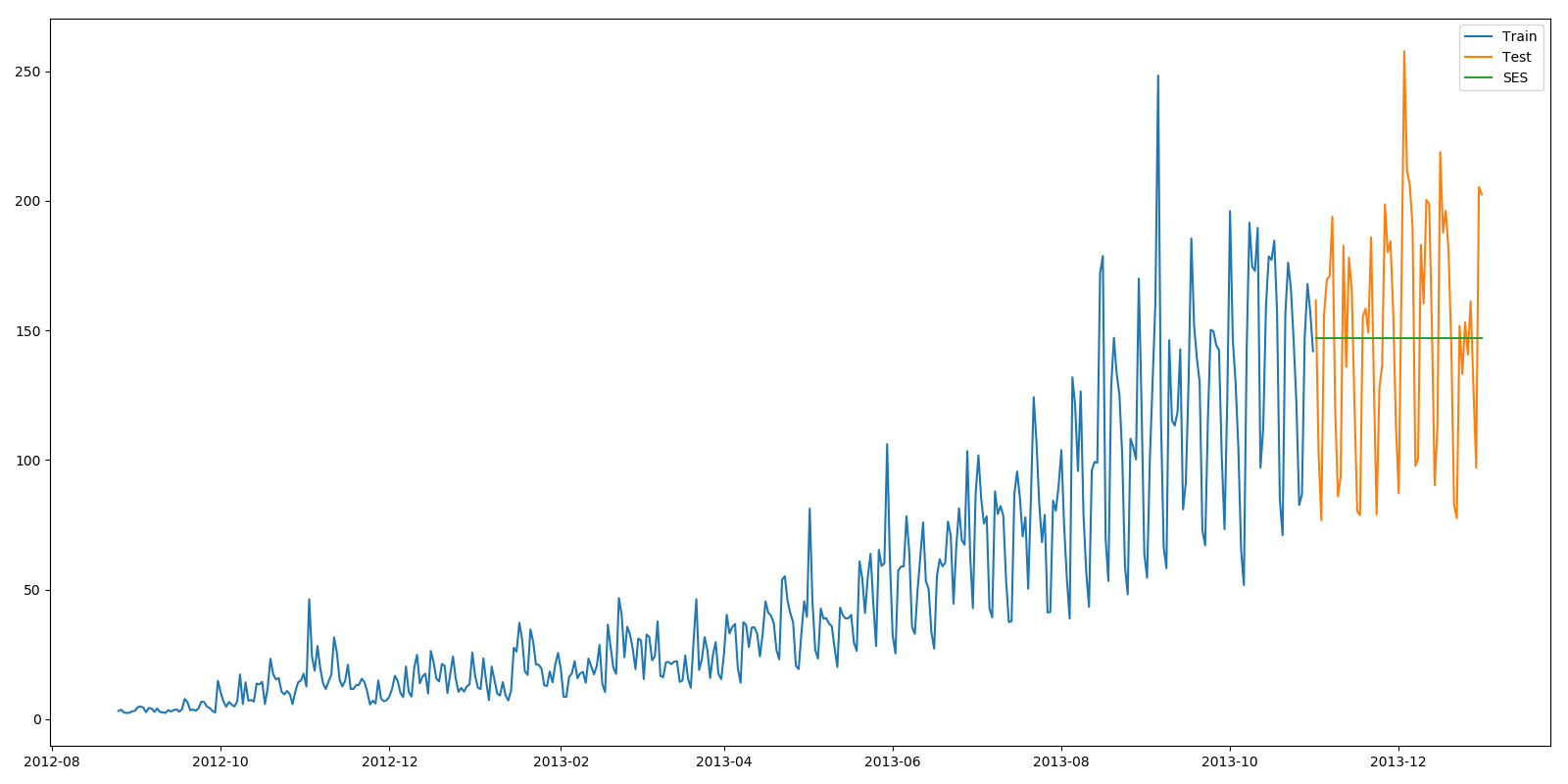from sklearn.metrics import mean_squared_error
from math import sqrt

rms = sqrt(mean_squared_error(test['Count'], y_hat_avg['SES']))
print(rms)


## 方法5：霍尔特(Holt)线性趋势法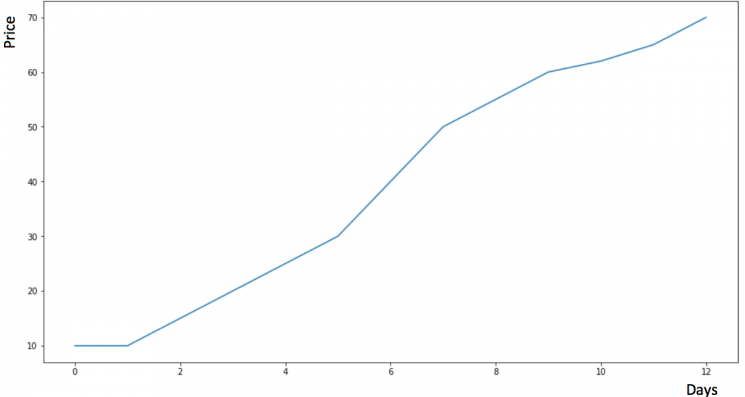import statsmodels.api as sm

sm.tsa.seasonal_decompose(train['Count']).plot()
plt.show()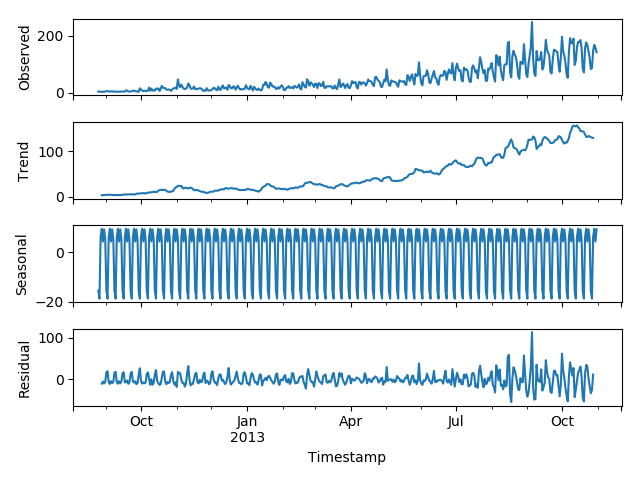$$\hat{y}_{t+h|t}=\iota _{t} + hb_{t}$$

$$\iota _{t}=\alpha y_t+(1-\alpha )(\iota _{t-1}+b_{t-1})$$

$$b_t=\beta *(\iota _t-\iota _{t-1})+(1-\beta )b_{t-1}$$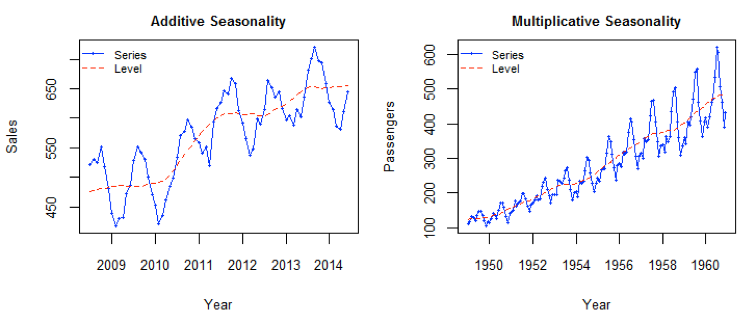from statsmodels.tsa.api import Holt

y_hat_avg = test.copy()

fit = Holt(np.asarray(train['Count'])).fit(smoothing_level=0.3, smoothing_slope=0.1)
y_hat_avg['Holt_linear'] = fit.forecast(len(test))

plt.figure(figsize=(16, 8))
plt.plot(train['Count'], label='Train')
plt.plot(test['Count'], label='Test')
plt.plot(y_hat_avg['Holt_linear'], label='Holt_linear')
plt.legend(loc='best')
plt.show()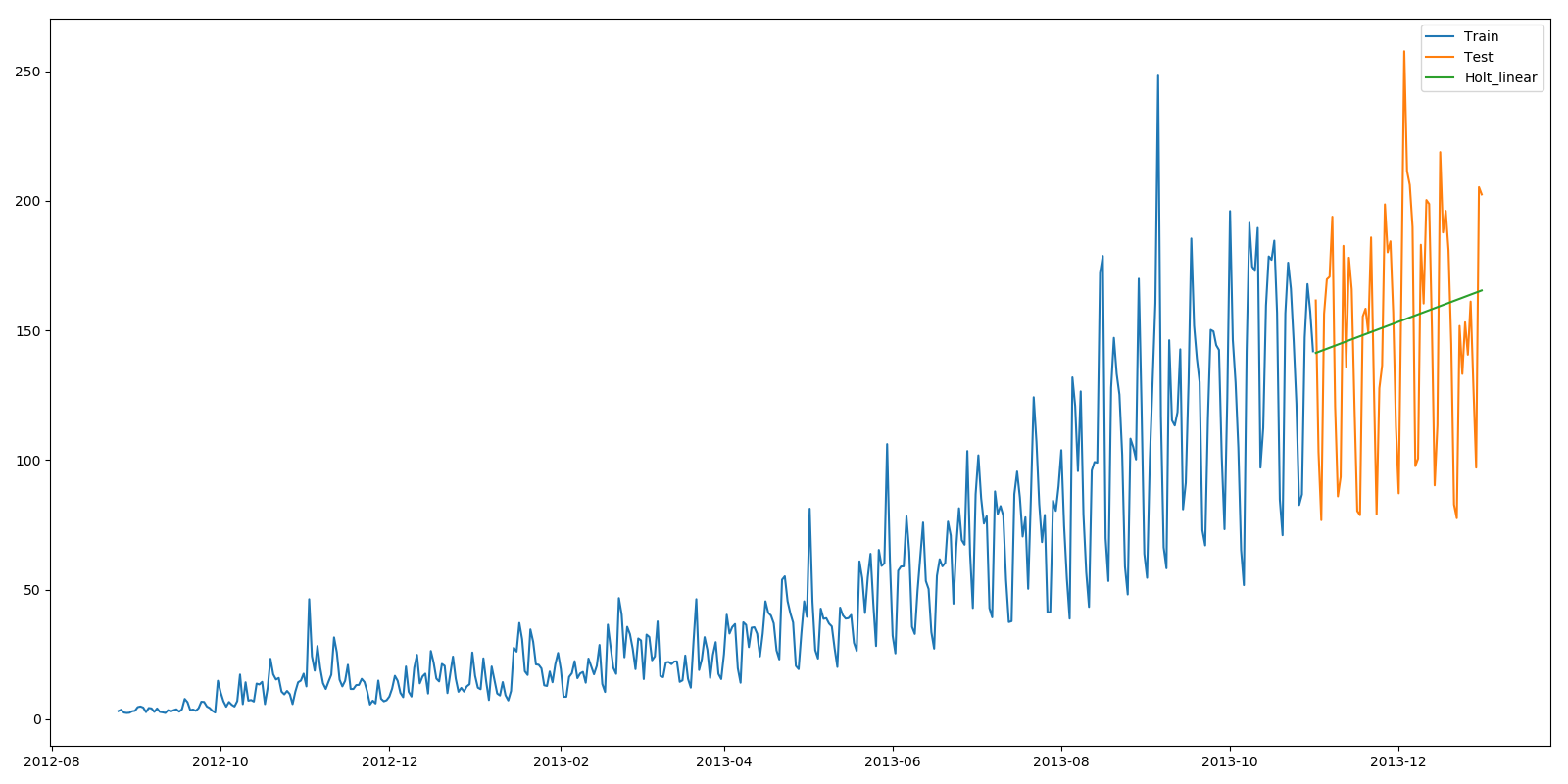from sklearn.metrics import mean_squared_error
from math import sqrt

rms = sqrt(mean_squared_error(test['Count'], y_hat_avg['Holt_linear']))
print(rms)


## 方法6：Holt-Winters季节性预测模型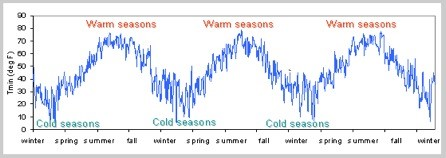Holt-Winters季节性预测模型由预测函数和三次平滑函数——一个是水平函数$ℓ_t$，一个是趋势函数$b_t$，一个是季节分量 $s_t$，以及平滑参数α,β和γ。

$$L_t = \alpha (y_t-S_{t-s})+(1-\alpha )(L_{t-1}+b_{t-1})$$

$$b_t = \beta (L_t-L_{t-1})+(1-\beta )b_{t-1}$$

$$S_t = \gamma (y_t-L_t)+(1-\gamma )S_{t-s}$$

$$F_{t+k} = L_t +kb_t +S_{t+k-s}$$

from statsmodels.tsa.api import ExponentialSmoothing

y_hat_avg = test.copy()
y_hat_avg['Holt_Winter'] = fit1.forecast(len(test))
plt.figure(figsize=(16, 8))
plt.plot(train['Count'], label='Train')
plt.plot(test['Count'], label='Test')
plt.plot(y_hat_avg['Holt_Winter'], label='Holt_Winter')
plt.legend(loc='best')
plt.show()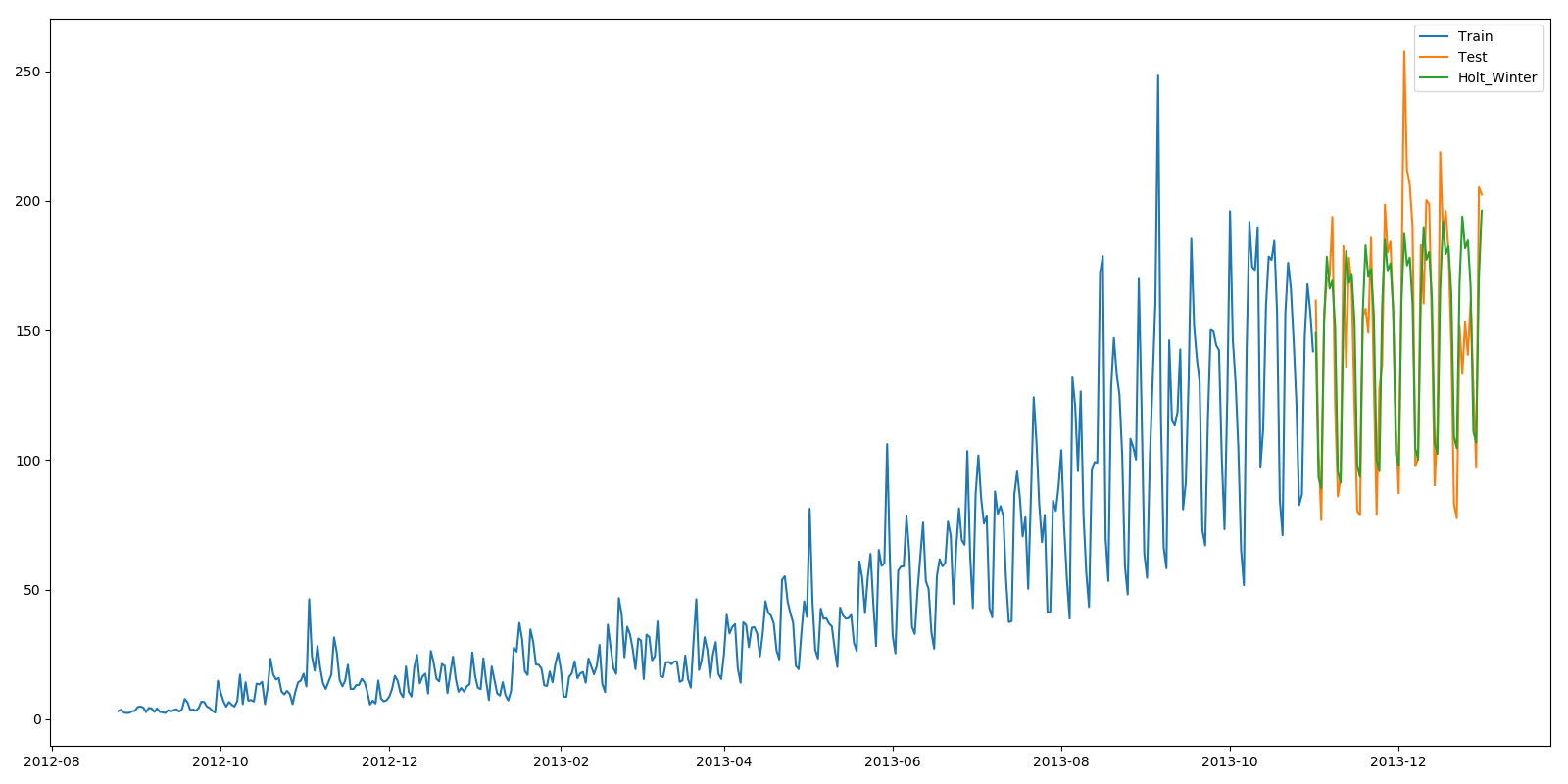from sklearn.metrics import mean_squared_error
from math import sqrt

rms = sqrt(mean_squared_error(test['Count'], y_hat_avg['Holt_Winter']))
print(rms)


## 方法7：自回归移动平均模型（ARIMA）

import statsmodels.api as sm

y_hat_avg = test.copy()
fit1 = sm.tsa.statespace.SARIMAX(train.Count, order=(2, 1, 4), seasonal_order=(0, 1, 1, 7)).fit()
y_hat_avg['SARIMA'] = fit1.predict(start="2013-11-1", end="2013-12-31", dynamic=True)
plt.figure(figsize=(16, 8))
plt.plot(train['Count'], label='Train')
plt.plot(test['Count'], label='Test')
plt.plot(y_hat_avg['SARIMA'], label='SARIMA')
plt.legend(loc='best')
plt.show()from sklearn.metrics import mean_squared_error
from math import sqrt

rms = sqrt(mean_squared_error(test['Count'], y_hat_avg['SARIMA']))
print(rms)


## 总结##### Windows+IntelliJ IDEA+Maven环境配置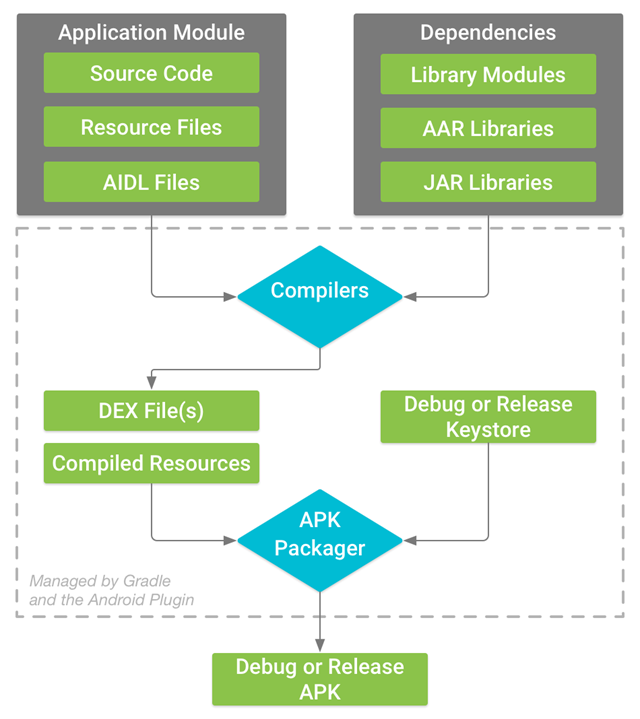##### Android应用：apk文件反编译## 2 Replies to “时间序列预测的7种方法”

1. chep说道：

可以尝试一些集成学习、深度学习算法

2. Aquark说道：

感谢作者分享！